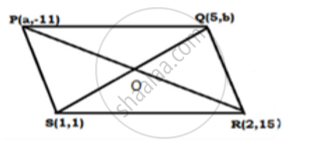# If the Points P (A,-11) , Q (5,B) ,R (2,15) and S (1,1). Are the Vertices of a Parallelogram Pqrs, Find the Values of a and B. - Mathematics

If the points P (a,-11) , Q (5,b) ,R (2,15)  and S (1,1). are the vertices of a parallelogram PQRS, find the values of a and b.

#### Solution

The points are  P (a,-11),Q(5,b) , R (2,15) and S(1,1).Join PR and QS, intersecting at O.

We know that the diagonals of a parallelogram bisect each other Therefore, O is the midpoint of PR as well as QS.

"Midpoint of PR" = ((a+2)/2,(-11+15)/2) = ((a+2)/2,4/2) = ((a+2)/2,2)

"Midpoint of QS " = ((5+1)/2 , (b+1)/2) = (6/2 ,(b+1)/2) = (3,(b+1)/2)

Therefore ,   (a+2)/2 =3, (b+1)/2= 2

⇒ a +2 = 6, b+1=4

⇒ a = 6 - 2,  b = 4-1

⇒ a = 4 and b= 3

Concept: Coordinate Geometry
Is there an error in this question or solution?

#### APPEARS IN

RS Aggarwal Secondary School Class 10 Maths
Chapter 16 Coordinate Geomentry
Q 25